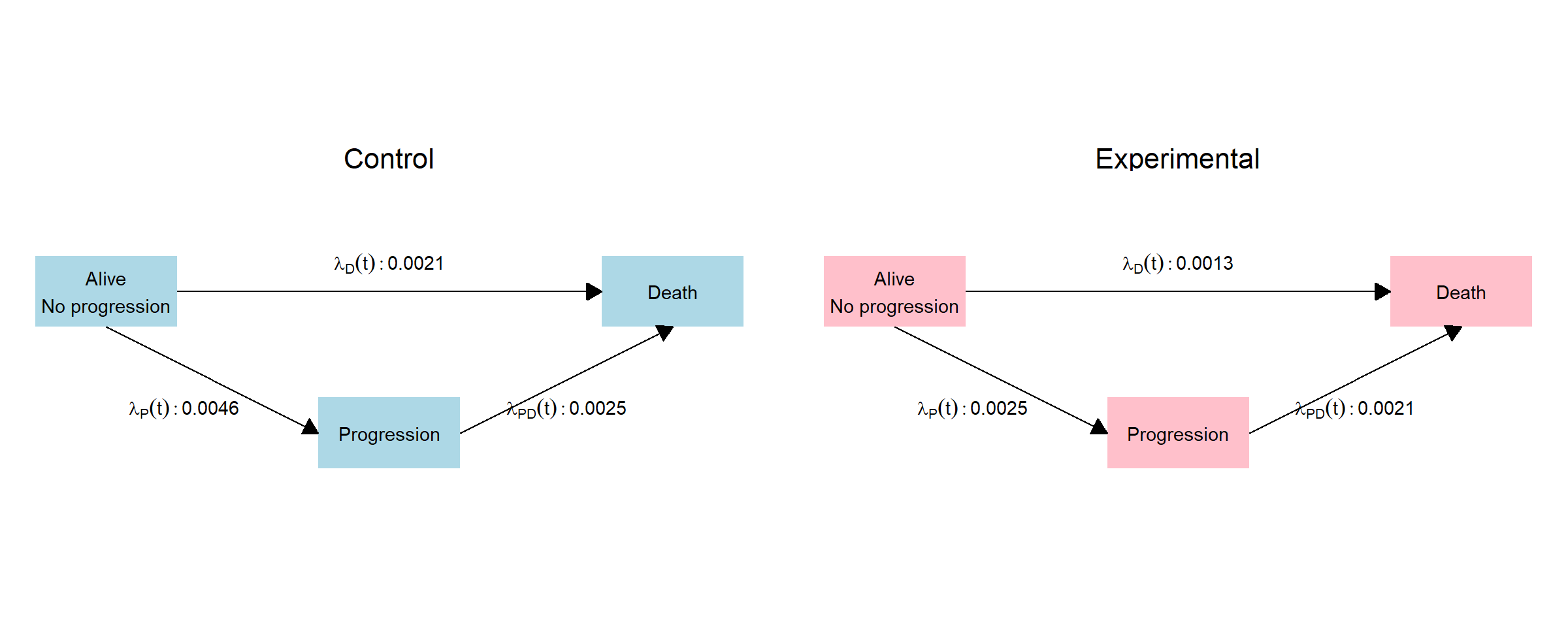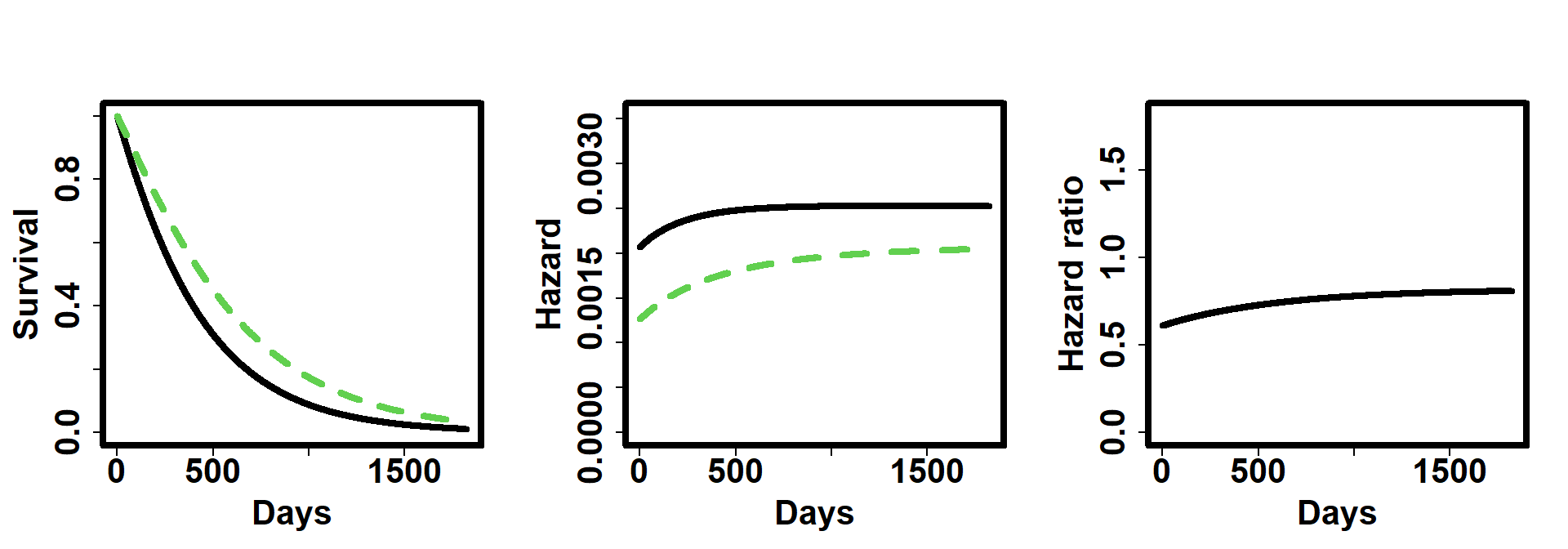In this vignette we showcase the various options to specify non-proportional hazards with the nph package.

``library(nph)``

Consider a clinical trial in which patients are randomised to either an experimental treatment or a control one. The considered endpoint is survival time and the follow-up time is 5-years.

# 1 Progression of disease

The first example we consider is non-proportional hazards due to the case when there is progression of disease that affects the survival of the patients. Moreover, since the progression appears with different rates for treatment and control arms, it leads to a violation of the non-proportional assumption.

As an example, consider the case where the median time to progression of disease is 9 montths for the experimental treatment (TRT) and 5 months for the control one (CTRL). Before progression, the median overall survival (OS) times are 18 (TRT) and 9 (CTRL) and after progression they decrease to 11 (TRT) and 9 (CTRL).

This is example is roughly based on the results observed in the study Keynote 189 (NEJM issue:22; 2018)

``````times <- c(0, 5 * 365)   # Time interval boundaries, in days

# Treatment group
t_resp <- 1              # There are no subgroups
B5 <- pop_pchaz(
T = times,
lambdaMat1    = m2r(matrix(18,nrow = 1)),
lambdaMat2    = m2r(matrix(11,nrow = 1)),
lambdaProgMat = m2r(matrix(9, nrow = 1)),
p = t_resp,
timezero = FALSE, discrete_approximation = TRUE
)

# Control group
c_resp <- 1              # There are no subgroups
K5  <- pop_pchaz(
T = times,
lambdaMat1    = m2r(matrix(11,nrow = 1)),
lambdaMat2    = m2r(matrix(9, nrow = 1)),
lambdaProgMat = m2r(matrix(5, nrow = 1)),
p = c_resp,
timezero = TRUE, discrete_approximation = TRUE
)

pp = plot_diagram(B5, K5)
pp``````The plot shows us the the survival and hazard functions by group, together with the hazard ratio. We observe that the HR is not constant through time and conclude that progression of disease leads to a non-proportional hazard.

``plot_shhr(K5, B5)``# 2 Subgroup with differential effect

Another feature that may lead a violation of the non-proportional hazards assumption is the presence of predictive subgroup. That is, subgroups with a differential treatment effect. We then build on the previous example to include a subgroup (with prevalence of 20%) with an additional benefit. The median time to progression in the subgroup is 15mo, while the median OS is 30mo before progression and 20mo after progression. For the complement of the subgroup, the parameters are maintained at the values of the previous example.

``````times <- c(0, 5 * 365)   # Time interval boundaries, in days

# Treatment group
t_resp <-  c(.2, .8)
B5 <- pop_pchaz(
T = times,
lambdaMat1    = m2r(matrix(c(30,
18),nrow = 2)),
lambdaMat2    = m2r(matrix(c(20,
11),nrow = 2)),
lambdaProgMat = m2r(matrix(c(15,
9), nrow = 2)),
p = t_resp,
timezero = FALSE, discrete_approximation = TRUE
)

# Control group
c_resp <- 1
K5  <- pop_pchaz(
T = times,
lambdaMat1    = m2r(matrix(11,nrow = 1)),
lambdaMat2    = m2r(matrix(9, nrow = 1)),
lambdaProgMat = m2r(matrix(5, nrow = 1)),
p = c_resp,
timezero = TRUE, discrete_approximation = TRUE
)

pp = plot_diagram(B5, K5, A_subgr_labels = c("S1", "S2"))
pp``````